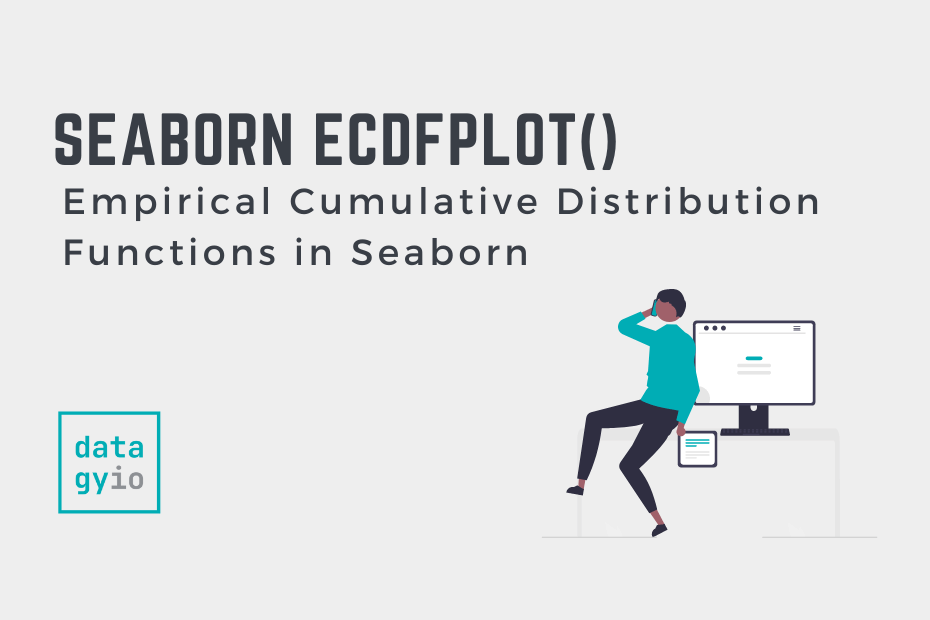# Seaborn ecdfplot – Empirical Cumulative Distribution FunctionsIn this guide, you’ll learn how to use the Seaborn `ecdfplot()` function to create empirical cumulative distribution functions (ECDF) to visualize the distribution of a dataset. ECDF plots are valuable tools to visualize how datasets are distributed, allowing you to gain strong insight into your data. In this tutorial, you’ll learn about the different parameters and options of the Seaborn ecdfplot function.

By the end of this tutorial, you’ll have learned the following:

• How the Seaborn `ecdfplot()` function works
• How to customize your Seaborn ECDF plots using color

## Understanding the Seaborn ecdfplot Function

Before diving into creating histograms in Seaborn, let’s explore the `sns.ecdfplot()` function. Take a look at the code block below to see the various parameters the function has to offer:

``````# Understanding the ecdfplot Function
seaborn.ecdfplot(data=None, *, x=None, y=None, hue=None, weights=None, stat='proportion', complementary=False, palette=None, hue_order=None, hue_norm=None, log_scale=None, legend=True, ax=None, **kwargs)``````

We can see that the function offers a large variety of parameters. While this tutorial won’t cover all of them, you’ll learn about the most important ones, including:

• `data=` provides the data to plot via a Pandas DataFrame
• `x=` and `y=` provide the variables to plot on the x- and y-axis respectively
• `hue=` adds an additional variable to plot via a color mapping

Now that you have a good understanding of the parameters the `sns.ecdfplot()` function offers, let’s dive into creating histograms.

## Creating a Seaborn ECDF Plot with ecdfplot

In order to create a simple Empirical Cumulative Distribution Function using Seaborn, we can pass a Pandas DataFrame and a column label into the `sns.ecdfplot()` function. For this, we can use the `data=` parameter for the DataFrame and the `x=` parameter for the column label.

Let’s see how we can accomplish this in Python:

``````# Creating a ECDF Plot in Seaborn
import seaborn as sns
import matplotlib.pyplot as plt

sns.ecdfplot(data=df, x='body_mass_g')
plt.show()``````

In the code block above, we imported the `'penguins'`dataset and then used the `'body_mass_g'` column label for the x-axis argument. This returns the image below:

We can see that this produces a stepped cumulative distribution, which extends from 0% to 100% of the dataset’s points. While similar to a cumulative histogram or KDE plot, the ECDF plot doesn’t hide any details.

## Creating a Horizontal ECDF Plot in Seaborn

We can easily create a horizontal ECDF plot in Seaborn by passing a column label into the `y=` parameter. This will rotate the plot so that the data expands horizontally, rather than vertically.

Let’s see how we can create a horizontal ECDF plot in Seaborn:

``````# Creating a Horizontal ECDF Plot in Seaborn
import seaborn as sns
import matplotlib.pyplot as plt

sns.ecdfplot(data=df, y='body_mass_g')
plt.show()``````

In the code block above, we used the same code as in our previous example. However, we switched the `x=` parameter for the `y=` parameter. In this case, the distribution’s values are plotted along the y-axis and the proportion is plotted along the x-axis.

In the following section, you’ll learn how to plot multiple groups of data in Seaborn ECDF plots.

## Plotting Multiple Groups in Seaborn ECDF Plots with Hue

Seaborn allows you to add a color semantic to ECDF plots using the `hue=` parameter. This allows you to pass in a column label by which to split the distribution. This will add a color for each unique value in that column, allowing you to better understand how the data for each category are distributed.

``````# Creating a ECDF Plot in Seaborn with Color
import seaborn as sns
import matplotlib.pyplot as plt

sns.ecdfplot(data=df, x='body_mass_g', hue='sex')
plt.show()``````

In the code block above, we added `hue='sex'`, which split the data by that column. This allows us to see how the body mass varies by gender.

In the final section below, you’ll learn how to show counts rather than proportions in ECDF plots.

## Showing Counts Rather than Proportions in Seaborn ECDF Plots

By default, Seaborn will plot the proportion of values, rather than the counts. In order to show the counts of values rather than the proportion, use `stat='count'` as the argument. Let’s see what this looks like:

``````# Showing Counts Rather than Proportions
import seaborn as sns
import matplotlib.pyplot as plt

sns.ecdfplot(data=df, x='body_mass_g', hue='sex', stat='count')
plt.show()``````

In the example above, we passed count into the `stat=` parameter. This changes the axis to be counts, rather than proportions. You’ll also notice that the different colors now have different endpoints for where they end.

We can see how this gives us information not just on the distribution but also how many data points are in each category.

## Conclusion

In this guide, you learned how to use the Seaborn `ecdfplot()` function to create informative ECDF plots in Seaborn. ECDF plots allow you to get a strong understanding of the distribution of data.

You first learned what the Seaborn ecdfplot function offers in terms of parameters and default arguments. Then, you learned how to create simple ECDF plots. From there, you built on what you learned to create more complex and informative ECDF plots by adding colors, changing scales, and more.